# Activity analysis

A linear activity analysis model [a9] is specified by two non-negative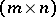-matrices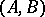, whereis the number of producible goods (assumed to be completely divisible) andis the number of possible processes or activities of production. The matrixis the input matrix:indicates the quantity of goodrequired as an input if theth activity is used at a unit level of intensity. The matrix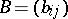is the output matrix: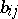is the quantity of goodproduced when theth activity is operated at unit intensity. The processes can be interpreted as "different ways of doing things" in a firm ([a2], [a3]) or different "sectors" of an economy ([a3], [a4]). It is assumed that these can be operated at any non-negative level. If a non-negative column vectordenotes the levels of operations, an output vector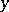is produced by using an input vectordefined as:A special case (simple linear model, [a2]) assumes that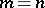(the number of goods and activities is the same) and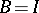, the-matrix identity (each process produces only one good).

A more general model [a5] is developed by defining an activity as a pair of non-negative-vectors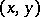where the output vectoris producible from the input vector. Assumptions are imposed on the structure of the set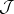of all activities (is called the technology or production possibility set of the firm or the economy).

A non-linear activity analysis model in which production is subject to random shocks is studied in [a7].

Some theoretical applications are sketched below.

### Production to maximize revenue given resource constraints.

Consider [a2] the simple linear modeland assume that the firm can sell a unit of goodat a price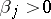. In the short run the firm has a fixed supply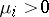of goodthat can be used as input. The problem is to choose a non-negative vector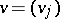(interpreted as the firm's production schedule) so as to maximize revenue subject to the constraints of resource availability; more formally,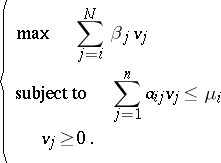Problems of this type have been solved by linear programming methods [a2].

### Planning for consumption targets.

Consider ([a2], [a5])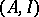, now interpreted as an economy withsectors, each producing a single good. Suppose that the sectors are operated at an intensity vector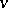. The output vector is given byand the input vector is. The net output available for consumption is. The problem of attaining a consumption target is posed as follows: Given any non-negative "target" consumption vector, does there exist a non-negativesuch that? The answer is "yes" if and only ifexists and is non-negative. A number of equivalent conditions are available ([a2], [a5]).

### Balanced growth at a maximal rate and the von Neumann equilibrium.

Consider ([a2], [a5], [a9]) an economy with a technology. Assume that:

i)is a closed, convex cone in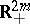;

ii)implies that(impossibility of free production);

iii)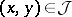,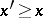,imply that(free disposal);

iv) for every commoditythere is some activity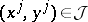such that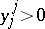(each good is produced by some activity). In view of i), the last assumption iv) can be equivalently stated as: there is awith a strictly positive. To understand von Neumann's problem of balanced growth of all goods, define the rate of expansion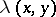of an activity, with a positive, as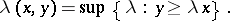One can show that under assumptions i)–iv) there is a maximal rate of expansion that the economy can attain. More formally, there are an activity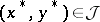, and asuch that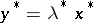,, and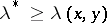for allwith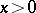. Moreover, there is a positive price system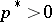such thatfor all. One refers to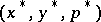as a von Neumann equilibrium. It has played a prominent role in characterizing "turnpike" properties of a class of finite horizon planning models [a8]. For the simple linear model, the existence of a von Neumann equilibrium can be studied by using the Perron–Frobenius theorem on the dominant characteristic root of a positive matrix [a5] (cf. also Frobenius matrix).

### Efficient allocation of resources.

The problem of attaining an efficient allocation of resources by using a decentralized system of decision making in which prices are used to coordinate individual decisions has long been of interest to economists (theorists as well as policy makers). Although the problem was first posed and solved in a static model (somewhat artificial, since "production" takes time), the subtleties that are involved were exposed in the context of an infinite horizon economy [a6].

Consider the simple linear modeland assume that each column ofis positive. Let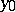be the strictly positive vector of initial stocks ofgoods. A program of resource allocation is a sequence of non-negative vectors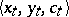satisfying,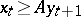for all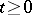. A programis efficient if there is no other program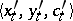from the same initialsuch thatfor all, and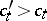for some. In other words,is efficient if there is no other program from the same initial stock that generates at least as much consumption of all goods in all periods, and strictly more of some good in some period.

One can show that for any programfrom a given,a program is efficient if and only if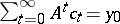.

The problem of optimal growth in the simple linear modelwhen one maximizes a welfare function over all programs from the initial stockis studied in [a1]. An account of the pioneering empirical work of W.W. Leontief involving activity analysis model is [a4].